##### Specific Latent Heat1. Figure 4.15 shows ice changing from solid to liquid when left at room temperature.

2. Figure 4.16 shows water turning into water vapour when boiling continues.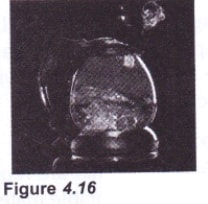3. While in Form 1, you have learned about the changes in the state of matter caused by the absorption and release of heat.

4. When ice melts or boils, heat is absorbed to overcome the attractive force or bonding between molecules. The heat absorbed is known as latent heat.

The latent heat, L, is the heat released or absorbed by a substance during the change of state at constant temperature.5. The molecules inside the solid are bound by very strong bonds. When heat energy is absorbed by ice at 0°C as shown in Figure 4.17, the heat energy is used to break up the bonds between molecules.

6. The absorbed heat energy is not converted to kinetic energy of the molecules, only the potential energy of the molecule is increased to change its state from solid to liquid. Therefore, the ice melts into water at 0°C.

7. The heat absorbed by the solid during the process of melting is known as latent heat of fusion.8. During boiling as shown in Figure 4.18, the heat absorbed by the boiling water at 100°C, is used to overcome the bonds between the molecule that hold them in liquid form. Water vaporises and change to steam at 100°C.

9. The heat absorbed by the liquid during vaporisation is known as the latent heat of vaporisation.

10. Figure 4.19. shows the heating graph for ice.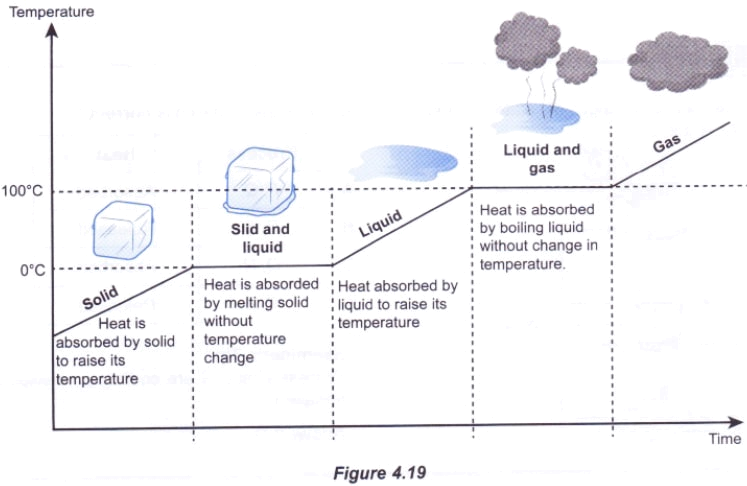11. Figure 4.20 shows the change of the potential energy, $${ E }_{ p }$$ with change of state. Kinetic energy, $${ E }_{ K }$$ changes with temperature.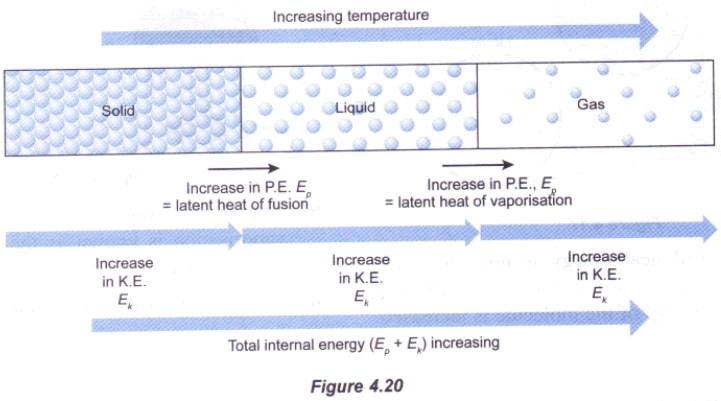Specific Latent Heat

1. If you need to melt a block of ice as shown in Figure 4.21, you need more latent heat because the mass is larger.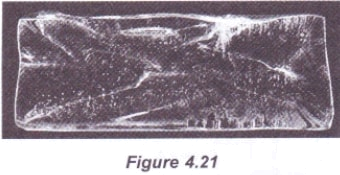2. The latent heat depends on mass and type of substance.

3. If you want to compare the latent heat in different types of substances, it is best to consider the latent heat per unit mass of the substance, that is, the specific latent heat.

Specific latent heat$$l$$, is the quantity of heat absorbed or released during the phase change of 1 kg of a substance without a change in temperature.

4. The S.I. unit for specific latent heat is $$J\ { kg }^{ -1 }$$. The equation for specific latent heat, $$l$$ is

$$l=\frac { Q }{ m }$$

where
$$Q=$$ Latent heat absorbed or released

$$m=$$ Mass of substance

The expression for the quantity of heat absorbed or released in as follows:

$$Q=ml$$

5. For phase changes between solids and liquids, the heat absorbed or released is known as specific latent heat of fusion$${ l }_{ f }$$, and it is defined as follows:

The specific latent heat of fusion$${ l }_{ f }$$ of a substance is the quantity of heat required to change the state of 1 kg of a substance from solid to liquid without a change in temperature.

6. Table 4.2 shows the melting point and specific latent heat of fusion for some substances.

 Substances Melting point °C Specific latent heat of fusion $${ J\ kg }^{ -1 }$$ Ice 0 $${ 3.34\times 10 }^{ 5 }$$ Butter 35 $${ 6.00\times 10 }^{ 4 }$$ Candle 57 $${2.10\times 10 }^{ 5 }$$ Sulphur 119 $${ 3.81\times 10 }^{ 4 }$$ Magnesium 650 $${ 3.73\times 10 }^{ 2 }$$

7. By comparing ice and candle, the candle with a higher melting point has smaller specific latent heat of fusion.

8. For the phase change between liquid and gas, the heat absorbed or released is known as specific latent heat of vaporisation, $${ l }_{ v }$$ and is defined as follows:

The specific latent heat of vaporisation, $${ l }_{ v }$$ of a substance is the quantity of heat required to change the state of 1 kg of a substance from liquid to gas without a change in temperature.

9. Table 4.3 shows the boiling point and specific latent heat of vaporisation for some substances.

 Substances Boiling point °C Specific latent heat of vaporisation $${ J\ kg }^{ -1 }$$ Ether 35 $${ 3.77\times 10 }^{ 5 }$$ Water 100 $${ 2.26\times 10 }^{ 6 }$$ Kerosene 210 $${2.51\times 10 }^{ 5 }$$ Sulphur 445 $${ 1.51\times 10 }^{ 6 }$$ Magnesium 1 107 $${ 5.31\times 10 }^{ 3 }$$

10. By comparing water with kerosene oil, the kerosene with a higher boiling point has a smaller specific latent heat of vaporisation.

Evaporation Causes Cooling Effect1. Figure 4.24 shows a boy feeling cold after leaving the pool. Why does this happen?

2. This is because the boy has a cooling effect caused by evaporation. When water evaporates, it carries away heat energy from the surface of the skin. Therefore, the boy feels cold.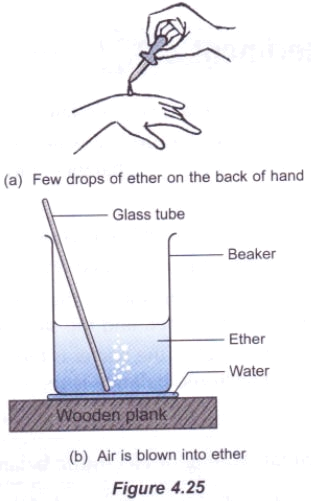3. When a few drops of ether on the hand as in Figure 4.25(a) is blown, the hand feels cold because when ether evaporates, it carries away the heat from the hand.

4. A beaker containing liquid ether fluid is placed over a layer of water on a wooden plank as shown in Figure 4.25(b). Air is blown into the ether to form air bubbles.

5. After the air is blown into the beaker containing ether, it is found that the beaker sticks to the wooden plank.

6. This is because the layer of water under the breaker has frozen into ice.

7. This situation is caused by the cooling effect which causes the water to turn into ice. Ether receives the latent heat that it needs to evaporate from the water and subsequently produces a cooling effect.

Applications of specific latent heat in daily life- Sweat evaporated from the skin has a cooling effect. When body temperature increases (during exercise), the sweat glands produce sweat. Specific latent heat of vaporisation is removed from the body when sweat evaporates and cools the body.- Steaming is a cooking process using steam. Continuos boiling water produces steam. Steam with high specific latent heat of vaporisation can provide a lot of heat. When steam is condensed onto food, a large quantity of latent heat is released directly into the food and allows it to cook quickly.- In the refrigerator, the liquified hydrofluorocarbon (HFC-134a), which is easily evaporated, is pumped through a set of pipes that are vented into the freezer. When hydrofluorocarbon fluids pass through the pipes this fluids absorbs heat from the refrigerator and evaporates. When hot hydrofluorocarbon gas passes through the compressor pump, the hot gas is compressed and converted back to liquid, eliminating the latent heat to the surrounding. This process is repeated to reduce the temperature in the refrigerator.